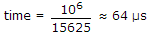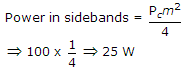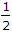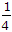# Online Electronics and Communication Engineering Test - Communication Systems Test 3Loading Test...

Instruction:

• This is a FREE online test. DO NOT pay money to anyone to attend this test.
• Total number of questions : 20.
• Time alloted : 30 minutes.
• Each question carry 1 mark, no negative marks.
• DO NOT refresh the page.
• All the best :-).

1.

In CCIR-B system, the time between start of one H syn. pulse and next is

A.
 64 μsB.
 6.4 μsC.
 640 μsD.
 0.64 μsExplanation:

The frequency of H pulses is 15625 Hz.

Hence.

2.

In a CD player the speed of CD is

A.
 constant at 500 rpmB.
 constant at 200 rpmC.
 varies from 200 to 500 rpmD.
 none of the aboveExplanation:

Since the circumference of outer tracks is more than that of tracks near the centre, the speed of disc is varied from 200 rpm to 500 rpm.

3.

In a 100% amplitude modulated signal, the power in the upper sideband when carrier power is to be 100 W and modulation system SSBSC, is

A.
 100 WB.
 66 WC.
 50 WD.
 25 WExplanation:

Modulation index = 100%1

Pc = 100 W4.

Trinitron is a monochrome picture tube.

A.
 TrueB.
 FalseExplanation:

Frequency band for local programs is fixed.

5.

Two sinusoidal signals of same amplitude and frequencies 10 kHz and 10.1 kHz are added together. The combined signal is given to an ideal frequency detector. The output of the detector is

A.
 0.1 kHz sinusoidalB.
 10.1 kHz sinusoidalC.
 a linear functionD.
 a constantExplanation:

Y(t) = A cos (2p x 104 t) + A cos (2p x 10.1 x 103 t)

when passed through high pass filter,

Output will be A cos (2p x 10.1 x 103 t) only.

6.

In cellular telephone each cell is designed to handle

A.
 45 two way communicationsB.
 90 two way communicationsC.
 45 one way communicationsD.
 180 two way communicationsExplanation:

Each cell is designed for 45 two way conversations.

7.

Which is a digital coding technique?

A.
 PCMB.
 DMC.
 DPCMD.
 All8.

The IF frequencies in a TV receiver

A.
 38.9 MHz for video and 33.4 MHz for audioB.
 38.9 MHz for both video and audioC.
 33.4 MHz for both video and audioD.
 33.4 MHz for video and 38.9 MHz for audio9.

In FDM systems used for telephone, which modulation scheme is adopted?

A.
 AMB.
 DSB-SCC.
 SSB-SCD.
 FM10.

Noise can be reduced by increasing sampling rate

A.
 trueB.
 falseC.
 can't sayD.
 insufficient data11.

In cell communication, cell stands for

A.
 Geographical AreaB.
 BatteryC.
 LocationD.
 No meaning12.

If the circumference of a spherical target is between 1 to 10 wavelength in a radar system, the situation is called

A.
 resonance regionB.
 Rayleigh regionC.
 optical regionD.
 binomial region13.

Square law modulators utilise

A.
 linear range of V-I characteristics of a diodeB.
 linear range of V-I characteristics of a triodeC.
 non-linear region of V-I dynamic characteristics of a diodeD.
 non-linear region of V-I dynamic characteristics of a triode14.

The maximum permissible distance between two samples of a 10 kHz signal

A.
 100 μ secB.
 50 μ secC.
 10 μ secD.
 none15.

In AM the amplitude of carrier components ... while in FM it

A.
 remains constant, does not remain constantB.
 does not remain constant ... remains constantC.
 remains constant... also remains constantD.
 does not remain constant, also does not remain constant16.

If carrier modulated by a digital bit stream had one of the possible phases of 0°, 90°, 180°, 270°, then the modulation is called.

A.
 BPSKB.
 QPSKC.
 QAMD.
 MSK17.

If F(ω) is Fourier transform of f(t), then Fourier transform of f(t) cos2 ωct is

A.F(ω) +[F(ω + 2ωc) + F(ω - 2ωc)]B.
 F(ω) +[F(ω + 2ωc) + F(ω - 2ωc)]C.
 F(ω) -[F(ω + 2ωc) + F(ω - 2ωc)]D.F(ω) -[F(ω + 2ωc) + F(ω - 2ωc)]18.

The currents fed to the two dipoles of turnstile antenna are

A.
 in phaseB.
 in quadratureC.
 in phase oppositionD.
 either (a) or (c)19.

Consider the following statements as regards BPSK and QPSK:

1. In BPSK we deal individually with each bit of duration Tb
2. In QPSK we lump two bits to form a symbol
3. In both BPSK and QPSK signal changes occur at bit rate
4. In QPSK the symbol can have any one of four possible values
Which of the above statements are correct?

A.
 1, 2, 3, 4B.
 1, 2, 3C.
 1, 2, 4D.
 2, 3, 420.

A pre-emphasis circuit provides extra noise immunity by

A.
 converting the phase modulation to frequency modulationB.
 pre-amplifying the whole audio bandC.
 boosting the base frequenciesD.
 amplifying the higher audio frequencies